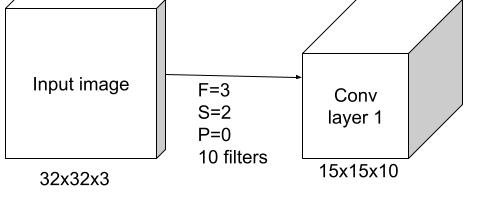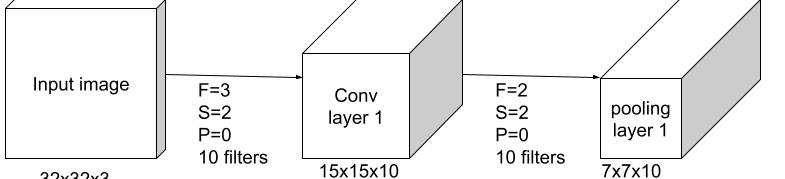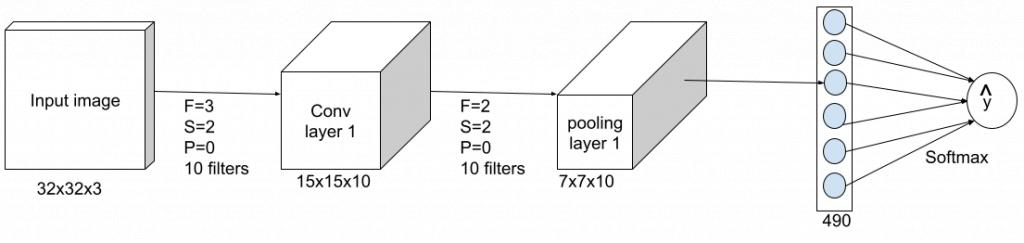CNN(Convolutional Neural Networks，卷积神经网络)，CNN在图像、提取空间信息中有着广泛的应用，CNN通常包含以下几种神经层：

# 1 卷积层的输出

• filter num：卷积核的数量
• filter size：卷积核的大小
• stride：卷积的步长

\begin{array}{c}
W_{output} = \frac{W_{input}-F}{S} + 1\\
H_{output} = \frac{H_{input}-F}{S} + 1\\
D_{output} = K
\end{array}

\begin{array}{c}
W_{output} = \frac{W_{input}-F+2P}{S} + 1\\
H_{output} = \frac{H_{input}-F+2P}{S} + 1\\
D_{output} = K
\end{array}

\begin{array}{c}
W = \frac{32-3+0}{2} + 1 = 15\\
H = \frac{32-3+0}{2} + 1 = 15\\
D = 10
\end{array}# 2 池化层的输出

\begin{array}{c}
W_{output} = \frac{W_{input}-F}{S} + 1\\
H_{output} = \frac{H_{input}-F}{S} + 1\\
D_{output} = K
\end{array}

\begin{array}{c}
W = \frac{15-2}{2} + 1 = 7\\
H = \frac{15-2}{2} + 1 = 7\\
D = 10
\end{array}# 3 全连接层的输出

\begin{array}{c}
outputsize = W_{input}\times H_{input}\times D_{input}
\end{array}

\begin{array}{c}
outputsize = 7 \times 7\times 10 = 490
\end{array}